## NCERT Solutions for Class 11 Maths Chapter 6 Linear Inequalities Exercise 6.3

You can find Chapter 6 Linear Inequalities Exercise 6.3 Class 11 Maths NCERT Solutions here which will guide in solving difficult questions. NCERT Solutions for Class 11 Maths are developed by subject matters of Studyrankers that are updated according to the latest pattern of CBSE. By practicing these questions, you will get to know about the types of questions that could come in the examination and how to solve them in less time.1. Solve the following systems of inequalities graphically:
x ≥ 3, y ≥ 2

x ≥ 3 ...(i)
and y ≥ 2 ...(ii)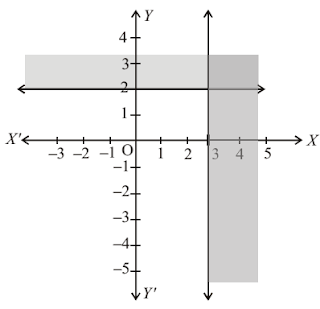Graph of inequality (i):
Let us draw the graph of the line x = 3. Clearly, x = 3 is a line parallel to y-axis at a distance of 3 units from the origin.
Since (0, 0) does not satisfy the inequality x ≥ 3 because 0 ≥ 3, is false. So the portion lying on the right side of x = 3 is the region represented by x ≥ 3.

Graph of inequality (ii):
Let us draw the graph of the line y = 2. Clearly, y = 2 is a line parallel to x-axis at a distance of 3 units from it. Since (0, 0) does not satisfy y ≥ 2 as 0 ≥ 2, which is false. So, the portion not containing the origin is represented by the given inequality.
The common region of the above two regions represents the solution set of the given linear constraints.

2. Solve the system of inequalities graphically:
3x + 2y ≤ 12, x ≥ 1, y ≥ 2

Inequalities are 3x + 2y ≤ 12, x ≥ 1, y ≥ 2
(i) The line 3x + 2y = 12 passes through (4, 0), (0, 6)
AB represents the line
3x + 2y = 12
Putting x = 0, y = 0 in
3x + 2y ≤ 12
0 + 0 ≤ 12
Which is true
∴ Origin lies in the region 3x + 2y ≤ 12 i.e 3x + 2y ≤ 12 represents the area below the line 3x + 2y = 12 and all the points lying on it.

(ii) The line x = 1 passes through (1, 0) and (1, 2). This line is represented by EF.
Consider the inequality x ≥ 1Putting x = 0, 0 ≥ 1 is not true
∴ Origin does not lie in the region
x≥ 1
The region on the right of EF.

(iii) The line y = 2, passes through the points (1, 2), (2, 2).
This line is represented by DC,
Putting y = 0 in y ≥ 2, we get 0 ≥ 2 , which is false.
Origin does not lie in this region, y ≥ 2 is represented by the region above y = 2 and all the points on this line. The region satisfying the inequalities.
3x + 2y ≤ 12, x ≥ 1, y ≥ 2 is the △EQR.

3. Solve the following systems of inequalities graphically
2x + y ≥ 6, 3x + 4y ≤ 12

2x + y ≥ 6 ...(i)
3x + 4y ≤ 12 ...(ii)
Graph of inequality (i): Let us draw the graph of the line
2x + y = 6
At y = 0, x = 3, we get the point (3, 0) on x-axis
At x = 0, y = 6, we get the point (0, 6) on y-axis.
Putting x = y = 0 in (i) we have 0 ≥ 6 which is false.
Hence, half-plane region not containing the origin is the solution region of the given inequality.

Graph of inequality (ii): Let us draw the graph of the line 3x + 4y = 12. At y = 0, x = 4 we get the point (4, 0) on x-axis.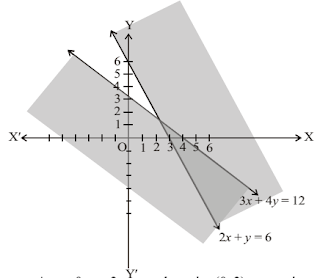At x = 0, y = 3 we get the point (0, 3) on y-axis.
Putting x = y = 0 in (ii) we have 0  ≤ 12 which is true.
Hence, half-plane containing the origin is the solution region of the given inequality.
The common region of the above two regions represents the solution set of the given linear system.

4. Solve the following systems of inequalities graphically
x + y > 4, 2x – y > 0

x + y > 4 ...(i)
2x – y > 0 ...(ii)
Graph of inequality (i):
Let us draw the graph of the line
x + y = 4 ...(iii)
At y = 0, x = 4 we get the point (4, 0) on x-axis
At x = 0, y = 4 we get the point (0, 4) on y-axis
Putting x = y = 0 in (i) we get 0 > 4 which is false.
Hence, half plane region not containing the origin is the solution region of the given inequality.

Graph of inequality (ii): Let us draw the graph of the line
2x – y = 0 ...(iv)
At x = 0 => 2(0) – y = 0 => y = 0
At x = 1 => 2(1) – y = 0 => y = 2
(0, 0) and (1, 2) are on the line (iv).
Draw a dotted line joining points (0, 0) and (1, 2).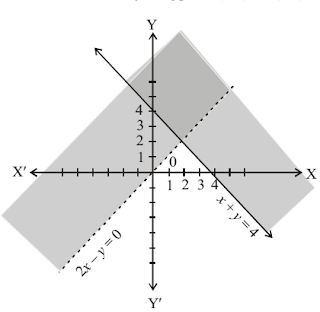To determine the region represented by the given inequality (ii), consider the point not lying on the line (iv), say (2, 1) and it lies in the half-plane of (ii) if 2(2) – 1 < 0, which is not true.
The half-plane region not containing (2, 1) represents the solution set of the given inequality. The common region of the above two regions represents the solution set of the given linear system.

5. Solve the system of inequalities graphically:
2x – y > 1, x – 2y < –1

2x – y > 1 … (i)
and x – 2y < –1 …(ii)
Graph of inequality (i):
Let us draw the graph of line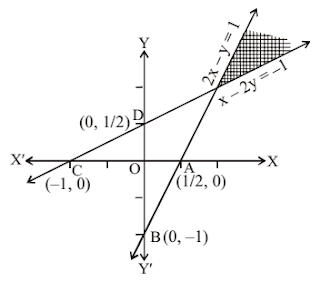2x – y = 1 … (iii)
at y = 0, x= 1/2, the point A is (1/2 , 0)
at x = 0, y = –1, the point B is (0, –1)
Putting x = y = 0 in (i), we have 0 > 1 which is false.
Hence, half plane region lying below AB is the solution region. Which is the shaded area. (not including the line AB)

Graph of inequality (ii):
Let us draw the graph of the line
x – 2y = –1 … (iv)
Putting y = 0, x = –1, point C is (–1, 0)
Putting x = 0, y = 1/2, point D is (0, 1/2)
Graph of (iv) is the line CD. Putting x = y = 0 in (ii) we have 0 < –1, which is false.
Hence, half plane region lying above CD is the solution area. Double shaded area is the solution region of the given inequalities.

6. Solve the following systems of inequalities graphically
x + y ≤ 6, x + y ≥ 4

x + y ≤ 6 ...(i)
x + y ≥ 4 ...(ii)
Graph of inequality (i): Let us draw the graph of the line x + y = 6.
At x = 0, x = 6, we get the point (6, 0) on x -axis.
At x = 0, y = 6, we get the point (0, 6) on y -axis.
Putting x = y = 0 in (i) we get 0 ≤ 6 which is true.Hence, half-plane containing the origin is the solution region of the given inequality.

Graph of inequality (ii): Let us draw the graph of the line x + y = 4.
At y = 0, x = 4 we get the point (4, 0) on x -axis.
At x = 0, y = 4 we get the point (0, 4) on y -axis.
Putting x = y = 0 in (ii) we get 0 ≥ 4 which is false. Hence half plane not containing the origin is the solution region of the given inequality.
The common region of the above two regions represent the solution set of the given linear constraints.

7. Solve the system of inequalities graphically:
2x + y ≥ 8, x + 2y ≥ 10

2x + y ≥ 8 ...(1)
and x + 2 y ≥ 10 ...(2)
Graph of inequality (1):
Let us draw the graph of the line
2x + y = 8 ...(3)
At y = 0, x = 4, we get
Point A(4, 0)
At x = 0, y = 8, we get
Point B (0, 8)Putting x = y = 0 in (1), we have 0 ≥ 8, which is false.
Hence, half plane region II lying above AB and including the line AB is the area of solution.

Graph of the inequality (2):
Let us draw the graph of line x + 2y = 10 ...(4)
At y = 0,
x = 10 Point C (10, 0) and
At x = 0, y = 5 Point D(0, 5)
Putting x = 0, y = 0 in (2), we have 0 ≥ 10, which is false.
Hence, upper portion of the line CD including the line CD is the shaded area. Double shaded area of figure is the solution region.

8. Solve the system of inequalities graphically
x + y ≤ 9, y > x, x ≥ 0

The inequalities and x + y ≤ 9, y > x, x ≥ 0
(i) The line x + y = 9 passes through (9, 0) and (0, 9). AB represents this line.
Putting x = 0, y = 0 in x + y ≤ 9
0 + 0 = 0 < 9
which is true
Origin lies in this region.Region below the line AB and all the points on it form the region x + y ≤ 9.

(ii) The line y = x, passes through the origin and (2, 2)
CD represents the line y = x
Putting x = 0, y = 1 in y – x > 0
1 – 0 > 0 which is true.
(0, 1) lies even in this region.
The inequality y > x is represented by the region above the line CD.

(iii) x > 0, (1, 0) lies in this region
The region x > 0 lies on the right of y axis Shaded region is the solution of
x + y ≤ 9, y > x, x ≥ 0

9. Solve the following systems of inequalities graphically
5x + 4y ≤ 20, x ≥ 1 y ≥ 2

5x + 4y ≤ 20 ...(i)
x ≥ 1 ...(ii)
y ≥ 2 ...(iii)
Graph of inequality (i): Let us draw the graph of the line
5x + 4y = 20
At y = 0 => 5x + 4 (0) = 20 => x = 4
At x = 0 => 5(0)+ 4y = 20 => y = 5
(4, 0) and (0, 5) are on the line (iv).
Putting x = y = 0 in (i) we get 5(0) + 4(0) ≤ 20
i.e., 0 ≤ 20 which is true.
Hence, half plane region containing the origin is the solution region of the given inequality.

Graph of inequality (ii): Let us draw the graph of the line x = 1 which is parallel to the y-axis and at a unit distance from it.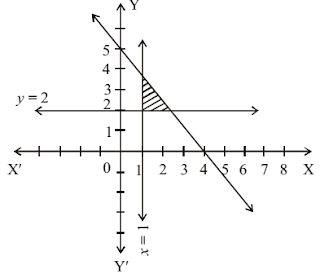Putting x = 0 in (ii), we have 0 ≥ 1 which is not true. Hence half-plane region not containing the origin is the solution region of the given inequality.

Graph of inequality (iii): Let us draw the graph of the line y = 2 which is parallel to x-axis and is at a distance of 2 units from it.
Putting y = 0 in (iii), we have 0 ≥ 2 which is not true.
Hence, half plane region not containing the origin is the solution region of the given inequality. The common region of the above three regions represents the solution set of the given linear system.

10. Solve the following systems of inequalities graphically
3x + 4y ≤  60, x + 3y ≤  30, x ≥ 0, y ≥ 0

3x + 4y ≤  60 ...(i)
x + 3y ≤  30 ...(ii)
x ≥ 0 ...(iii)
y ≥ 0 ...(iv)
Graph of inequality (i): Let us draw the graph of the line
3x + 4y = 60 ...(v)
At y = 0 => 3x + 4(0) = 60
x = 20
x = 0 => 3(0) + 4y = 60
=> y = 15
(20, 0) and (0, 15) are on the line (v)
Putting x = y = 0 in (i) we get 0 ≤  60 which is true.
Hence, half plane region containing the origin is the solution region of the given inequality.

Graph of inequality (ii): Let us draw the graph of the line
x + 3y = 30 ...(vi)
At y = 0 => x + 3 (0) = 30
=> x = 30
x = 0 => 0 + 3y = 30
=> y = 30
(30, 0) and (0, 30) are on the line (vi)
Putting x = y = 0 in (ii) we get 0 ≤  30 which is true. Hence, half plane region containing the origin is the solution region of the given inequality.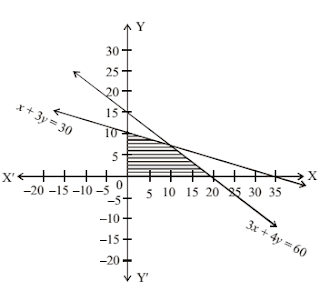Graph of inequality (iii): Clearly, x ≥ 0 represents the region lying on the right side of y-axis.

Graph of inequality (iv): Clearly, y ≥ 0 represents the region lying above the x-axis.
The common region of the above four regions is the solution set.

11. Solve the system of inequalities graphically:
2x + y ≥ 4, x + y ≤ 3, 2x - 3y ≤ 6

2x + y ≥ 4 … (i),
x + y ≤ 3 … (ii),
2x - 3y ≤ 6 …(iii)
We first draw the graph of lines 2x + y =4, x + y = 3 and 2x – 3y = 6
2x + y = 4, passes through A (2, 0) and B (0, 4)
Graph of it is ABSince, 0 >/ 4 origin does not lie in the region of 2x + y ≥ 4. This inequality represent the region above it.
Again, x + y = 3 is represented by the line CD, where C is (3, 0) and D is (0, 3).
Since 0 < 3, origin lies in the region of x + y ≤ 3
∴ x + y ≤ 3 represents the region below CD further 2x – 3y = 6 is represented by EC
where E is (0, –2) and C is (3, 0)
As 0 < 6, Origin lies in the region of
2x – 3y ≤ 6.
∴ 2x - 3y ≤ 6 represents the region below EC and all the points of EC
Triple shaded triangular area is the solution area in the solution region.

12. Solve the system of inequalities graphically
x – 2y ≤ 3, 3x + 4y ≥ 12, x ≥ 0, y ≥ 1

The inequalities are
x – 2y ≤ 3, 3x + 4y ≥ 12, x ≥ 0, y ≥ 1

(i) The line x – 2y = 3 passes through (3, 0) and (0 , -(3/2))
This is represented by AB. 0 < 3 denotes origin lies in the region x – 2y ≤ 3
∴ Region above this line and including its points represents x – 2y ≤ 3

(ii) 3x + 4y = 12 passes through (4, 0) and (0, 3).
CD represents this line.
Since 0 >/ 12. Origin does not lies in 3x + 4y ≥ 12 The region above CD and including points of CD represents 3x + 4y ≥ 12.

(iii) x ≥ 0 the region on the right of y-axis and all the points on it.

(iv) y = 1 is the line parallel to x-axis at a distance 1 from it.
y ≥ 1 or y – 1 > 0. Since – 1 >/ 0, origin does not lie in this region.
∴ y ≥ 1 is the region above y = 1 and the points lying on it.
The common shaded region is YC PQB which represents the solution the given inequalities.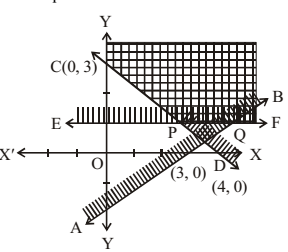13. Solve the systems of inequalities graphically
4x + 3y ≤ 60, y ≥ 2x, x ≥ 3, x, y ≥ 0

The inequalities are
4x + 3y ≤ 60, y ≥ 2x, x ≥ 3, x, y ≥ 0
(i) The line 4x + 3y = 60 passes through (15, 0), (0, 20) and it is represented by AB.
Putting x = 0, y = 0
∴ 3x + 4y ≤ 60
0 + 0 = 0 ≤ 60 which is true therefore, origin lies in this region. Thus, region below AB and the points lying on AB represent the inequality 4x + 3y ≤ 60.

(ii) y = 2x passes through (0, 0) and (5, 10). It is represented by CD.
Putting x = 0, y = 5 in y – 2x ≥ 0 (0, 5) lies in this region.Region lying above the line CD and including the points on CD represents y  2x.

(iii) x  3 is the region lying on the right of line x = 3 and points lying on x = 3 represent the inequality x  3
The shaded area is the PQR in which x > 0 and y > 0 is true for each point, is the solution of given inequalities.

14. Solve the given inequality 3x + 2y ≤ 150, x + 4y ≤ 80, x ≤ 15, y ≥ 0 graphically.

The inequalities are 3x + 2y ≤ 150, x + 4y ≤ 80, x ≤ 15, y ≥ 0
The line 3x + 2y ≤ 150 passes through the point (50, 0) and (0, 75). AB represent this line in the graph.
Putting x = 0, y = 0 in 3x + 2y ≤ 150 => 0 ≤ 150 shows that origin lies in this region(i) This region lying below AB and the points lying on AB represent the inequality 3x + 2y ≤ 150

(ii) The equation x + 4y ≤ 80 passes through the points (80, 0), (0, 20). This is represented by CD. Since 0 ≤ 80, A region lying below CD and the points of CD represent the inequality x + 4y ≤ 80

(iii) x ≤ 15 is the region lying on the left of EP. Thus the region X' PQC which is a double shaded region is the solution of the inequalities given.
It may be noted that any point lying in this region satisfies the inequality 3x + 2y ≤ 150

15. Solve the system of inequalities graphically
x + 2y ≤ 10, x + y ≥ 1, x – y ≥ 0, x ≥ 0, y ≥ 0

The inequalities are x + 2y ≤ 10, x + y ≥ 1, x – y ≥ 0, x ≥ 0, y ≥ 0
(i) x + 2y = 10 passes through (10, 0) and (0, 5).
The line AB represents this equation
∵ 0 ≤ 10.
∴ Origin lies in the region x + 2y ≤ 10.∴ Region lying below AB and the points lying on it represents x + 2y ≤ 10

(ii) x + y = 1 passes through (1, 0) and (0, 1). The line CD represents this equation 0 >/ 1. Origin does not lie on x + y ≥ 1.
∴ The region lying above the line CD and the points lying on CD represents the inequality x + y ≥ 1.

(iii) x – y = 0, passes through (0, 0) and (1, 1).
This is being represented by EF. Also (0, 1) lies on x – y ≤ 0.
The region lying above EF and the points lying on it represent the inequality x – y ≤ 0.

(iv) x ≥ 0 is the region lying on the right of y-axis and the points lying on y-axis.

(v) y ≥ 0 is the region above x-axis, and the points lying on y = 0.
The shaded area represents the inequalities given including y ≥ 0.